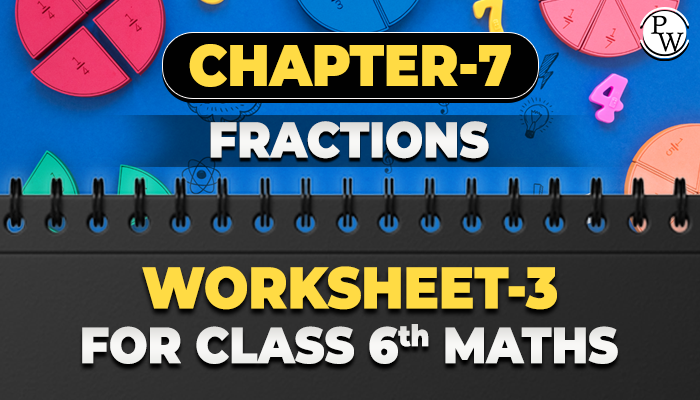# Chapter-7 Fractions Worksheet-3 for class 6 maths

#### Worksheets for Class 6 Maths

The academic team of Physics Wallah prepared worksheets and questions for chapter Fractions for all students who are in class 6. Physics Wallah questions for class 6 maths chapter Fractions consist of carefully selected numerical for class 6 maths and consist of step by step solutions of all questions with answers. Download worksheet-3 of class 6 maths chapter Fractions and start solving the questions for reference use the solutions.Before solving the worksheet of Physics Wallah it is highly recommended for reading the theory of the chapter and solving the questions given in your textbook. For additional information related to the subject, you can check the Maths Formula section. Do follow NCERT Solutions for class 6 Maths prepared by Physics Wallah.

## Solved Examples

Q1. Which of the following is the correct statement about fractions?
1. Fraction means one whole group
2. Fraction means a part of a group
3. Fraction is made only from prime numbers
4. All statements are correct

Ans. The correct option is 2

A small part of a large group or large number is called a fraction.

Q2. What is the numerator of 3/7?

1. 7
2. 1/7
3. 3
4. 1/3
Ans. Correct Option is 3

The numerator is the number above the line of the fraction

Q3. The equivalent fraction of 15/20 with denominator 80 is.
1. 60/80
2. 65/80
3. 70/80
4. 30/80
Ans.  For denominator 80, the fraction will be

15/20 x 4/4=60/80

Q4. Rohit exercised for 3/6 of an hour, while Manu exercised for 3/4 of an hour. Who exercised for a longer time?
1. Rohit
2. Manu
3. Both of them exercised for the same duration
4. None of them
Ans. Correct option is 2

Manu exercised for 3/4 of an hour, i.e., 45 minutes, while Rohit exercised for 3/6 or 1/2

Q5. Which of the following is the correct definition of Like Fractions?
1. Fractions that do not have the same denominator.
2. Fractions that have the same denominator.
3. Fractions that have the same numerator.
4. None of them.

Ans. Correct option is 2

Like fractions have the same denominators.

### Class 6 Maths Chapter-7 Fractions download your free pdf copy from the below-mentioned link.